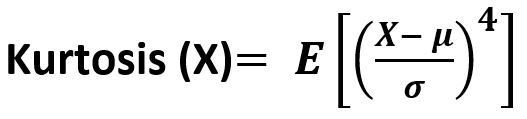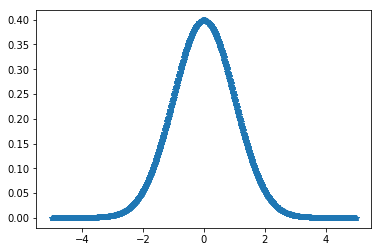# scipy stats.kurtosistest() function | Python

`scipy.stats.kurtosistest(array, axis=0)` function test whether the given data set has normal kurtosis (Fisher or Pearson) or not.

What is Kurtosis ?
It is the fourth central moment divided by the square of the variance. It is a measure of the “tailedness” i.e. descriptor of shape of probability distribution of a real-valued random variable. In simple terms, one can say it is a measure of how heavy tail is compared to a normal distribution.

Its formula –Parameters :
array : Input array or object having the elements.
axis : Axis along which the kurtosistest is to be computed. By default axis = 0.

Returns : Z-score (Statistics value) and P-value for the normally distributed data set.

Code #1:

 `# Graph using numpy.linspace()  ` `# finding kurtosis ` ` `  `from` `scipy.stats ``import` `kurtosistest ` `import` `numpy as np  ` `import` `pylab as p  ` ` `  `x1 ``=` `np.linspace( ``-``5``, ``5``, ``1000` `) ` `y1 ``=` `1.``/``(np.sqrt(``2.``*``np.pi)) ``*` `np.exp( ``-``.``5``*``(x1)``*``*``2`  `) ` ` `  `p.plot(x1, y1, ``'*'``) ` ` `  ` `  `print``( ``'\nKurtosis for normal distribution :\n'``, kurtosistest(y1)) `

Output :

```Kurtosis for normal distribution :
KurtosistestResult(statistic=-2.2557936070461615, pvalue=0.024083559905734513)
```
My Personal Notes arrow_drop_upCheck out this Author's contributed articles.

If you like GeeksforGeeks and would like to contribute, you can also write an article using contribute.geeksforgeeks.org or mail your article to contribute@geeksforgeeks.org. See your article appearing on the GeeksforGeeks main page and help other Geeks.

Please Improve this article if you find anything incorrect by clicking on the "Improve Article" button below.

Improved By : Akanksha_Rai

Article Tags :

Be the First to upvote.

Please write to us at contribute@geeksforgeeks.org to report any issue with the above content.